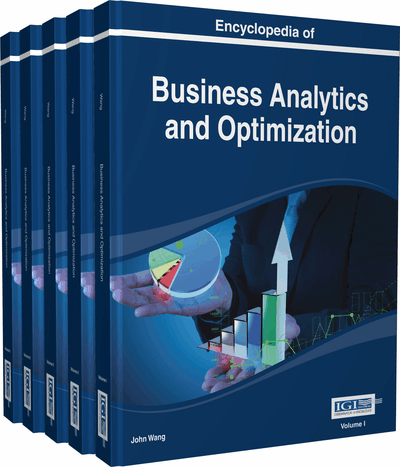# Bender Decomposition

Georgios K. D. Saharidis (University of Thessaly, Greece)
DOI: 10.4018/978-1-4666-5202-6.ch027

Top

## Introduction

In worldwide, the business environment becomes more and more complex making the solution of real case studies increasingly difficult due to the need to solve large-scale mathematical models. In a new age of big data and ever-evolving environment, managers and researchers need access to the most updated information about the trends in large-scale optimization. Business Analytics and Optimization are a group of approaches that help organizations understand and develop new insights of business performance, based on different methods and data. They make extensive use of data, statistical and qualitative analysis and fact-based management to drive decision making. They have been increasingly recognized by organizations as an important tool for large-scale optimization.

Large-scale optimization problems refer to optimization problems which are difficult to be solved to optimality. Large-scale optimization problems do not only depend on the number of parameters, decision variables and constraints. Frequently, even if these features are moderate, optimization problems could be still considered as large-scale due to complicating structures rendering them difficult to solve by the current optimization methods.

It has been more difficult the last decades to model and solve real case studies due to more complex systems and the massive data connecting these sub-systems. One of the best practices in order to optimize business analytics is to identify the best optimization approach in order to manage, analyze and use the available massive data. Because of the competitive environment and the need for Big Data sharing, the resulted mathematical model could not be solved to optimality without using a decomposition method. Thus, at the age of big data, we can use decomposition techniques to solve real size mathematical models and optimize the operation of a real-life system by e.g. avoiding an unpleasant outcome, decreasing operations cost or increasing the overall profit.

In real-life case studies, two types of decomposition techniques are applied: a) structural decomposition techniques and b) mathematical decomposition techniques. Structural decomposition aims at decomposing the initial large-scale system examined into two or more sub-systems based on a series of decomposition rules, which depend on the constraints of the system under study. After the structural decomposition of the initial system, the resulting sub-systems are optimized separately providing the optimal solution of the initial system, under specific conditions. The structural decomposition rules result, analysising the system under two optimization frameworks: a) centralized optimization and b) decentralized optimization (Saharidis, Dallery, & Karaesmen, 2006; Saharidis, Kouikoglou, & Dallery, 2009a). Mathematical decomposition aims at decomposing the initial large-scale mathematical model into two or more sub-models based on mathematical programming theorems, as for example, the duality theorem. In principle, the mathematical decomposition techniques do not depend on the case study but on the structure of the developed mathematical model, which describes the system under study. Mathematical decomposition techniques are: a) Benders decomposition (Saharidis, Minoux, & Ierapetritou, 2010), b) Dantzig-Wolfe decomposition (Dantzig & Wolfe, 1960) c) Lagrangian relaxation, d) Lagrangian decomposition and e) Cross decomposition.

In this chapter we focus on the Benders decomposition method that has been used in the past and is still used in our days in order to solve large-scale problems. The basic idea of decomposition methods is to decompose the initial large-scale optimization problem into sub-problems of smaller and more manageable size getting rid of the part of the problem that makes optimality hard to reach.

In the following section, we present an overview of more recent results and applications on the Benders decomposition technique. In next section, we define and present in details the concept of the Benders decomposition technique as well as the issues, controversies and problems arising when using and applying the Benders decomposition algorithm. Finally, in the last section of the chapter, we will discuss future and emerging trends on the Benders decomposition.

## Key Terms in this Chapter

Low Density Benders Cut: A low density Benders cut is a cut involving a small number of decision variables of RMP therefore its contribution to strengthening the RMP tends to be limited.

Valid Inequality: An inequality which significantly restrict the solution space of a problem without excluding the optimal solution and leading to improve the convergence of a solution approach.

Benders Decomposition: Benders decomposition method is a classical approach for combinatorial optimization problems, based on the idea of partition and constraint generation.

Block-Decomposed Mathematical Model: A mathematical model which can be decomposed into sub-models.

Multiple Constraints Generation Idea: The generation of more than one cut in each iteration of Benders algorithm.

Decomposition Technique: Solution method in which the main idea is to decompose the problem into sub-problems which are simpler to be solved.

Quality of Bender Cut: A measurement of how efficient Benders cut is, affecting the Benders algorithm convergence.

## Complete Chapter List

Search this Book:
Reset